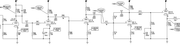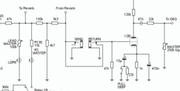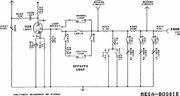## Some theoretical questions about tubes

All about tubes, in amplifiers and in stompboxes.
POTL
Resistor Ronker
Information
Posts: 331
Joined: 26 Sep 2016, 03:11
Has thanked: 58 times
Been thanked: 67 times
Hello
I had a few trivial questions about tube preamps. I understand that the forum is dedicated to stompboxes, but I am sure that competent people will help me.
I looked at the scheme of the preamp EVH 5150 III, which is a modernized version of the Peavey 5150/6505.
I have a few questions
1) In most preamplifier circuits, there is a voltage divider in front of the triode; however, before the V5-A, the r66 + r67 design resembles the input circuit of vintage amplifiers, what is called such a connection, what are its pros and cons, how can the influence of resistors on the volume drop be calculated (in the divider voltage can always be calculated on how many decibels volume falls).
2) V6-A is Triode with Local Negative Feedback, as far as I understand the gain is 1, and the bandwidth of the frequencies is very high, the input impedance is high and the output is low. A logical question arises: what advantage does this construction have with a classic buffer? Buffer is easier to perform and requires less detail, just so no one would complicate the scheme.
3) V6-B is a buffer, why is it needed if its functions are performed by the previous stage?4) Do resistors R7 and R8 affect the gain of V2-A or the nominal does not play a big role?
For example, if I change the value of 270 to 470, the gain will be less?5) Do the resistor values ​​in the voltage divider affect the gain?
For example, a pair of R47K + R47K and a pair of R470K + R470K will give the same voltage drop of 6dB or will the resistance value further affect the overall gain?
6) In Mesa Boogie Mark amplifiers, the output stage looks very interesting, there are several voltage dividers at once, which give a strong signal drop, what is this for?ppluis0
Diode Debunker
Information
Posts: 890
Joined: 14 Jul 2010, 18:33
Location: Argentina
Has thanked: 122 times
Been thanked: 284 times
POTL wrote:Hello
I had a few trivial questions about tube preamps. I understand that the forum is dedicated to stompboxes, but I am sure that competent people will help me.
I looked at the scheme of the preamp EVH 5150 III, which is a modernized version of the Peavey 5150/6505.
I have a few questions
1) In most preamplifier circuits, there is a voltage divider in front of the triode; however, before the V5-A, the r66 + r67 design resembles the input circuit of vintage amplifiers, what is called such a connection, what are its pros and cons, how can the influence of resistors on the volume drop be calculated (in the divider voltage can always be calculated on how many decibels volume falls).
R66 is to provide a ground reference for the triode grid. R67 is called "grid stopper" and this purpose is to create a low pass filter in conjunction with the input capacitance at the grid of that tube.

Info on this matter is in: https://www.ampbooks.com/ Look at the section "Amplifier calculators"
POTL wrote:2) V6-A is Triode with Local Negative Feedback, as far as I understand the gain is 1, and the bandwidth of the frequencies is very high, the input impedance is high and the output is low. A logical question arises: what advantage does this construction have with a classic buffer? Buffer is easier to perform and requires less detail, just so no one would complicate the scheme.
You find an extensive explanation at this link: http://www.valvewizard.co.uk/localfeedback.html
POTL wrote:3) V6-B is a buffer, why is it needed if its functions are performed by the previous stage?In this section there is an attenuator and tone shaping network composed by R74, R75, R76 and C31 Also the output taken at the cathode of V6-B has low impedance to drive the tone stack
POTL wrote:4) Do resistors R7 and R8 affect the gain of V2-A or the nominal does not play a big role?
For example, if I change the value of 270 to 470, the gain will be less?
[ Image ]
5) Do the resistor values ​​in the voltage divider affect the gain?
For example, a pair of R47K + R47K and a pair of R470K + R470K will give the same voltage drop of 6dB or will the resistance value further affect the overall gain?
These resistors are to mix the signal from each volume pot into a grid of the following tube and their value is related to the potentiometers involved.
POTL wrote:6) In Mesa Boogie Mark amplifiers, the output stage looks very interesting, there are several voltage dividers at once, which give a strong signal drop, what is this for?

[ Image ]

[ Image ]
The components represented as SPST and labelled as LDR13, 14, and 15 are photocells to select different channels or set of controls in those amps and open and close one at time.
There is not an big voltage divider as can be interpreted as first sight.

Cheers,
Jose